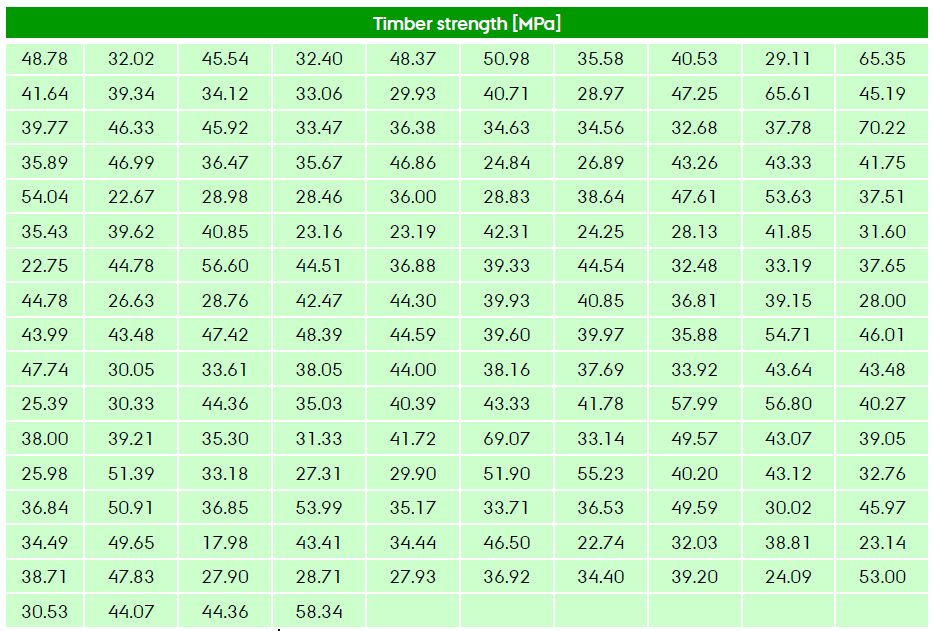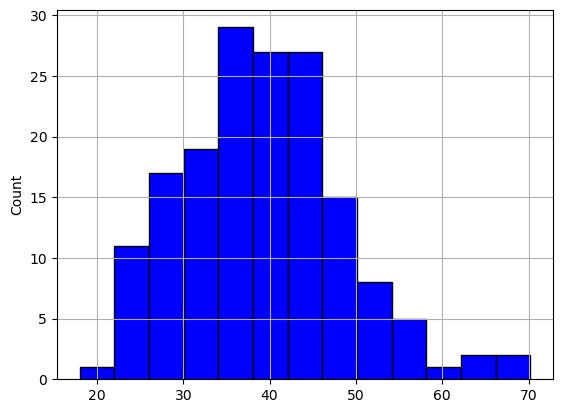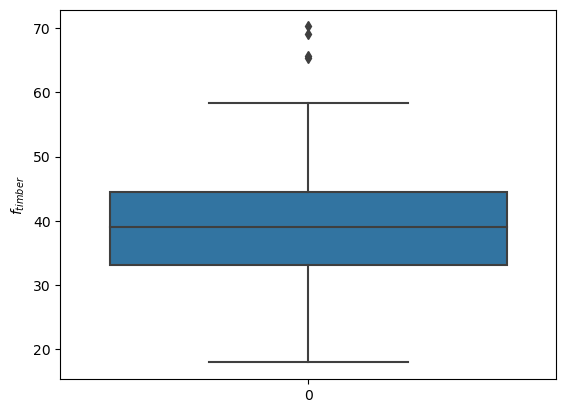top of page
Search

# Stat Data1: Data Analysis - timber strength 164 data

Updated: 3 days ago

We have a dataset of 164 data, timber strength.The data have some variability. This is expected because timber is a material naturally variable.

The focus here is Exploratory Data Analysis (EDA) of the dataset:

• statistics

• histograms

• cdf

• boxplot

At first, we import the main libraries that we will use

#------------------------

#Import modules

#------------------------

1 import numpy as np 2 from OpenAIUQ import Stat as sta In Line 1 we import numpy, the prefix for the module Stat is sta.

#--------------------------------------------------------------

#load the dataset (164 data of timber strength)

#--------------------------------------------------------------

1 dataset=np.loadtxt('timber164.txt') 2 #d is the object collecting the dataset 3 d=sta.data1(dataset) 4 data=d.data 5 d.disp_summary(content='reduced')

6 #d is the object data1

7 #d.data collect the data

8 #d.summary collects the summary

In Line 1 we load the dataset, like an ndarray (164,). In Line 3 we associate the object d (of sta.data1) to the dataset. To check the main statistical properties of the sample in Line 5 we use the method disp.summary of d.It is seen that the data range from min=17.98 MPa to a max=70.22 MPa. This implies that a good range analysis can be xx=[0, 80] MPa. The sample mean is m=39.32 MPa, the sample coefficient of variation v=24.02% confirms the high degree of variability. Skewness g1=0.53 shows that the data are a dominant right tail (this is good property for the strength of a material). Sample kurtosis g2=3.61 (greater than Gaussian) shows that the tails are heavy, this means that highest values of timber are not unlikely.

For a most clear representation, we plot the histograms

#--------------------------------------------------------------

#plot histograms

#--------------------------------------------------------------

1 d.plot_hist()Two main parameters of the method plot_hist of data1 are "stat" and "bins". The default values are stat='count' and bins='auto'. The value stat='count' means that we are represent the number of occurrencies in each class. Other choices are stat='probability' (which represents the relative frequencies) and stat='density' which normalizes the histograms to a Probability Density Function (pdf). Moreover, the binning is automatic. To address a more aware binning choices we write the following code:

1 edges=np.arange(0,85,5) 2 d.plot_hist(bins=edges,stat='probability')

3 #bins=edges: limits of the bins 4 #stat='probability': frequency at each bin

5 #d.fig is the figure

6 #d.ax is the subplot 7 d.ax.set_xlim(0,80) 8 d.ax.set_xticks(edges) 9 d.ax.set_xlabel('\$f_{timber} \ MPa\$'); 10 d.ax.set_title('Timber strength n=164')In Line 1 we are defining the array edge, ranging from f=0 MPa to f=80 MPa with a step delta=5 MPa. It represents the edges of the bins. In Line 2 we are plotting again the histograms, this time with chosen values of bins and in terms of relative frequency. The method plot_hist of data1 returns as output the attributes fig and ax. We use the attribute ax to define the limits of x-axis (Line 7), the ticks of x-axis (Line 8), the label of x-axis (Line 9) and the title of the figure (Line 10)

This binning shows a lack of data in the class [60,65] MPa, so maybe larger widths might be considered. However, we have explored different sizes of bin and the chosen histograms provide the same qualitative description of the data. The histograms show that the mode in the class [35.40] MPa (collecting also mean and median values), high variability around the mean value (coefficient of variation v=24.02%), longer tail right (g1=0.53, positive skewness) and heavy tails (kurtosis g2=3.61)

A more robust description of the dataset is given by the empirical distribution, provided by the method plot_cdf_emp of sta.data1.

#--------------------------------------------------------------

#plot empirical cdf

#-------------------------------------------------------------- 1 d.plot_cdf_emp() 2 #d.fig is the figure

3 #d.ax is the subplot

4 d.ax.set_xlim(0,80) 5 d.ax.set_ylim(0,1) 6 d.ax.set_xlabel('\$f_{timber} \ MPa\$'); 7 d.ax.set_ylabel('ecdf \$f_{timber}\$') 8 d.ax.set_title('Timber strength n=164')In line 1 we plot the ecdf, while in Lines 4-10 we edit the figure using the attribute ax of the object d. Since here we are analyzing a material strength, it may be of interest to evaluate the characteristic value

#--------------------------------------------------------------

#Characteristic value

#--------------------------------------------------------------

1 d.get_quantile(0.05) 2 print('f_k= ',d.Q,' Mpa')

In Line 1 we evaluate the quantile 5% of the dataset through the method get_quantile(p) of sta.data1. Its value is stored in the attribute Q of sta.data1. In Line 2 we print the value of the characteristic value.

Another used representation is the boxplot provided by the method plot_box of sta.data1

#--------------------------------------------------------------

#-------------------------------------------------------------- 1 d.plot_box()

2 #d.fig is the figure

3 #d.ax is the subplot 4 d.ax.set_ylabel('\$f_{timber}\$')The minimum and maximum values of the box are the lower interquartile Q1 (p=25%) (collected in d.Q1) and the upper interquartile Q3(p=75%) (collected in d.Q3). The central line of the box is the median Q2(p=50%) (collected in d.Q2). The boxplot describes also the limits of the dataset min=Q1-1.5*(Q3-Q1) and max=Q1+1.5*(Q3-Q1). Values beyond these lines are considered outliers. In Lines 4 we edit the label of the y-axis of the figure using the attribute ax of the object d.

timber164
.txt

timber164
.ipynb